# How to update data without resetting the axis limits

Hi This is my ScottPlot version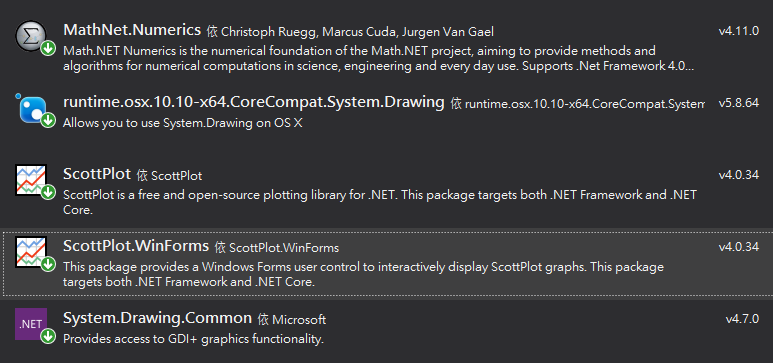I have no idea to disable automatic fit when keep render. I hope to check the signal details while chart drawing. But the plot will keep fit to data automatic. Like below FFT_X: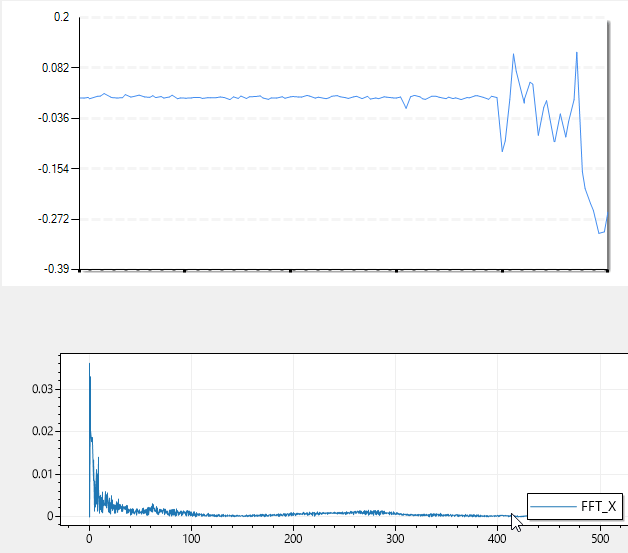I hope the effect is like your previous project Realtime Microphone FFT with ScottPlot.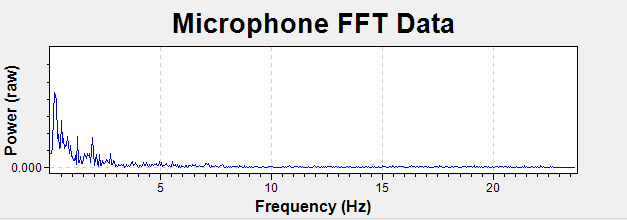Hope to achieve the effect by laste ScottPlot v4.0.34, Instead of `ScottPlot.ScottPlotUC` in previous project.

This is what I have tried:

``````cs
double[] fft_result = this.my_fft(src_wave);
var plt = this.chart_fft.plt;
plt.Clear();
plt.PlotSignal(fft_result, sample_rate, label: "FFT_X") ;
plt.Legend();
this.chart_fft.Render();
``````

• 点赞
• 写回答
• 关注问题
• 收藏
• 复制链接分享
• 邀请回答

#### 7条回答

• Hi

Hi I'm happy to see you got this working! I'm curious to know a little more about your application. What signal are you measuring?

The signal source is acceleration from accelerometer. sample rate is 1k hz. I'm planning to analyze motor vibration. And determine "how health" of the motor.

why is the size of your array changing? ... It seems like your input signal is a fixed size, so the FFT output (periodogram / power spectral density) should also be a fixed size.

Actually I do not fixed the input signal size. I designed to allow users choose the range to calculate FFT. It may be 1 second, 10 seconds, or other range, depends on the analysis needs.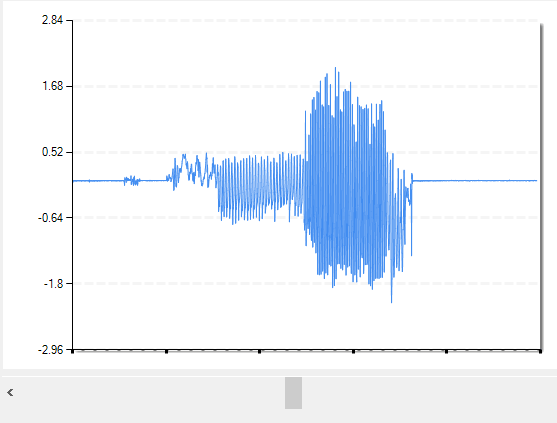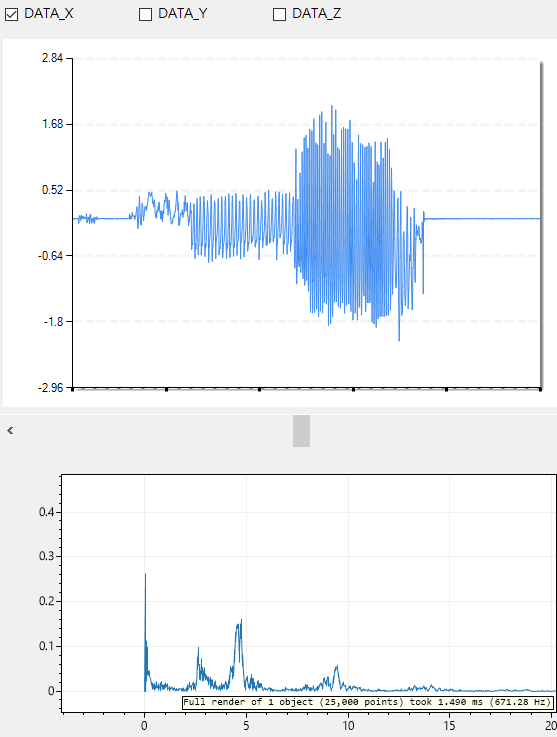Thanks for your reminder about performance. Maybe I will start check the performance impact of non-fixed signal.

BTW This is my tool for calculating FFT `MathNet.Numerics` gist I am also very interested in your `Spectrogram` project.

点赞 评论 复制链接分享
• Actually I do not fixed the input signal size. I designed to allow users choose the range to calculate FFT. It may be 1 second, 10 seconds, or other range, depends on the analysis needs.

Ah, that makes sense. Thanks for sharing the animated screenshots - this is a really cool application! I can see from the benchmark performance is fantastic so it looks like what you're doing is working great.

The gist is also helpful in reviewing how MathNet.Numerics handles FFT

Thanks for sharing!

点赞 评论 复制链接分享
It helped me a lot, and achieve the effect I want.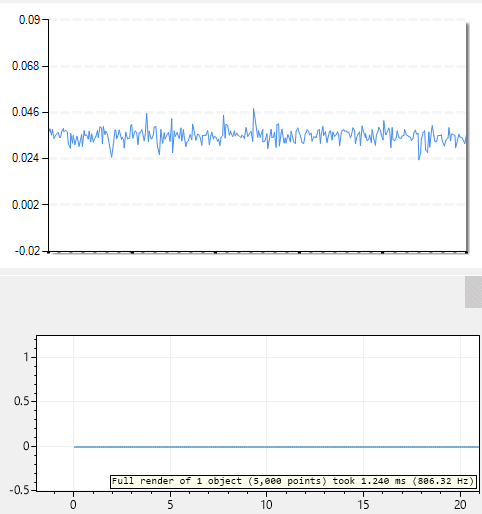But I encountered some problems in the process of writing program. When the length of `ys` or value of `sampleRate` change.

I am follow suggest 1, initial the formsPlot

``````cs
double[] sin = ScottPlot.DataGen.Sin(50, 2);
this.fft_plt_signal = this.chart_fft.plt.PlotSignal(sin, 1000);
this.chart_fft.Render();
``````

Result like below.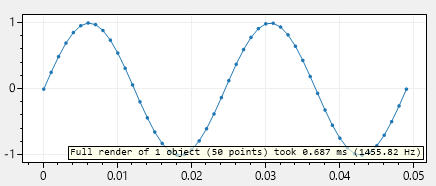and then update internal `ys` in animation loop.

``````cs
this.fft_plt_signal.ys = my_fft(src_wave);
this.chart_fft.Render();
``````

`chart_fft` only shows part of the result. Even if the `ys` number has increased to 1558.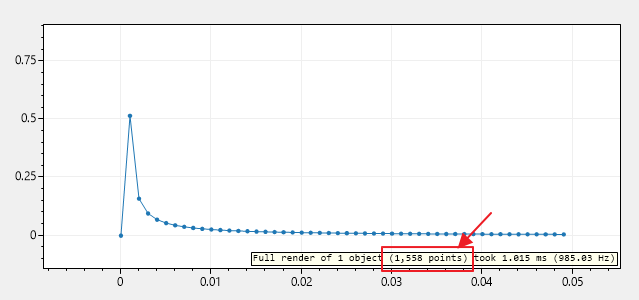So I modify `maxRenderIndex` to fit `ys.length`.

``````cs
this.fft_plt_signal.ys = my_fft(src_wave);
this.fft_plt_signal.maxRenderIndex = this.fft_plt_signal.ys.Length; // here
// this.fft_plt_signal.maxRenderIndex = this.fft_plt_signal.ys.Length - 1; // Maybe this is the correct
this.chart_fft.Render();
``````

I am not sure this way is correct, but now the `formsPlot` can render all the `ys`.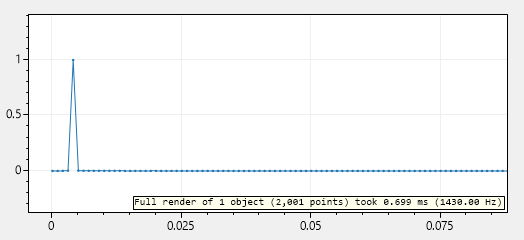Last I want to change the `sampleRate`

``````cs
this.fft_plt_signal.ys = my_fft(src_wave);
this.fft_plt_signal.maxRenderIndex = this.fft_plt_signal.ys.Length;
this.fft_plt_signal.sampleRate = 1.0; // here
this.chart_fft.Render();
``````

But the x axis tick value has not changed. Correctly it should become 0 - 5000. When `point number = 5000, sampleRate = 1`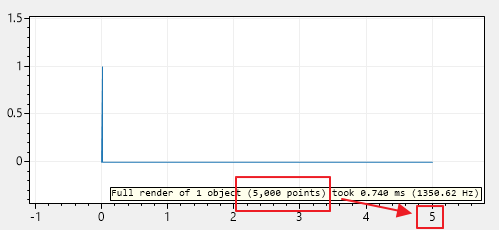I must also change `samplePeriod` when update `sampleRate`

``````cs
this.fft_plt_signal.ys = my_fft(src_wave);
this.fft_plt_signal.maxRenderIndex = this.fft_plt_signal.ys.Length;

this.fft_plt_signal.sampleRate = 1.0;
this.fft_plt_signal.samplePeriod = 1.0 / this.fft_plt_signal.sampleRate; // here

this.chart_fft.Render();
``````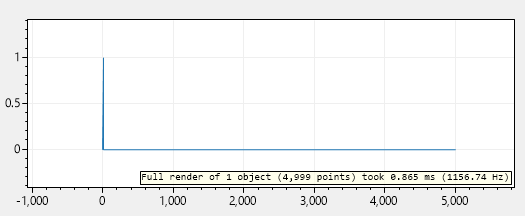Maybe these two values can change at same time by encapsulation. I think it can be modified like below: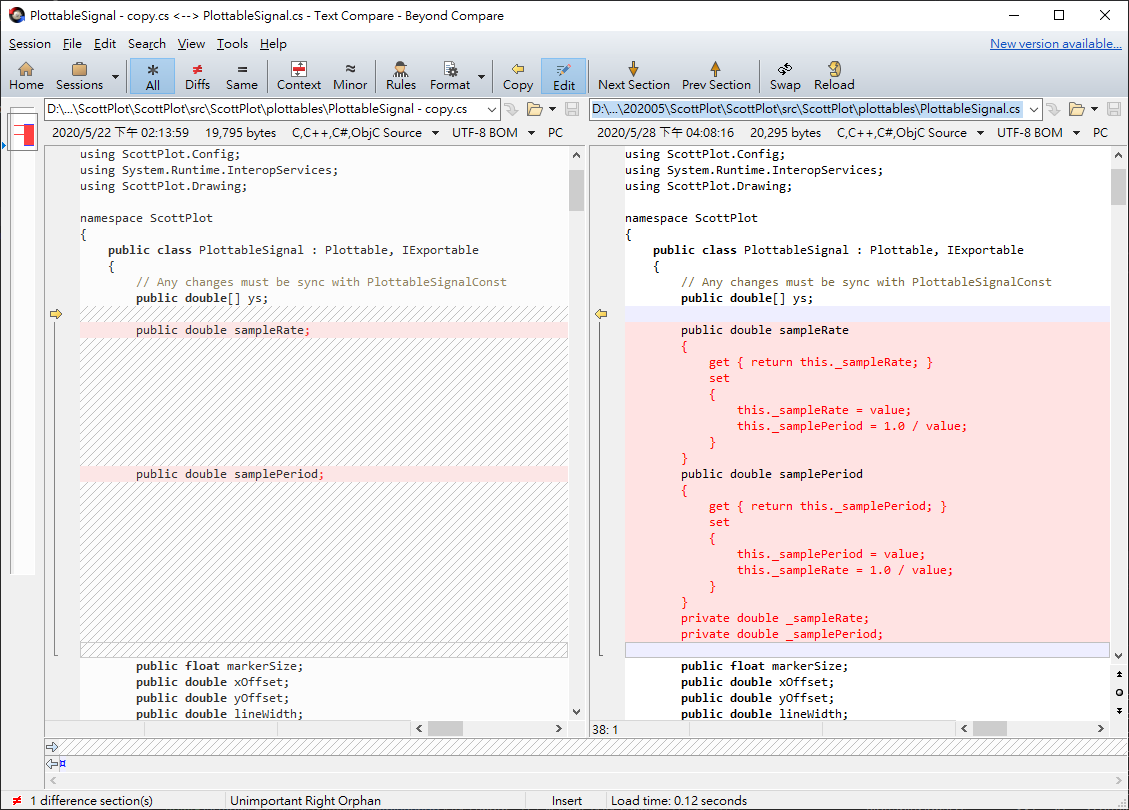Thanks a lot.

点赞 评论 复制链接分享
It helped me a lot, and achieve the effect I want.

Hi I'm happy to see you got this working! I'm curious to know a little more about your application. What signal are you measuring?

Coincidentally I've been working on two FFT-related projects this week: * https://github.com/swharden/FftSharp * https://github.com/swharden/Spectrogram

I can't help but ask - why is the size of your array changing? You could get a huge performance improvement if you don't create a whole new array in memory with every frame you draw on the screen. It seems like your input signal is a fixed size, so the FFT output (periodogram / power spectral density) should also be a fixed size. Even if your input array is "growing" (less than the expected size) it can be padded with zeros prior to calculating the FFT so all arrays are fixed-size and don't have to be recreated and garbage collected frequently. So, while your current solution works, I wanted to suggest double-checking to make sure all those `new` statements you must be doing are really necessary. Further, if your input array size really is changing with every iteration, your FFT algorithm may be zero-padding your input data anyway to achieve a fixed size, since the FFT algorithm requires an input array with a length that is an even power of 2. Just a thought!

### Updating sampleRate and Sample Period

But I encountered some problems in the process of writing program. When the length of `ys` or value of `sampleRate` change.

Thanks for mentioning this! The problem, as you found, is that `samplePeriod` is calculated in the initializer and is not properly updated if you update `sampleRate`.

A good solution, as suggested by your screenshot, is to use properties so updating one updates the other too. This is a very good idea!

### maxRenderIndex

Similarly, you noted that `maxRenderIndex` is being calculated (and matched to `ys.Length`) in the initializer, and this doesn't account for a situation where the user sets a differently sized `ys` later. The best solution here is to move this size check to inside the render loop.

Thank you for these suggestions, and the excellent animated screenshots. They are very helpful! I'll implement these ideas this weekend.

点赞 评论 复制链接分享
• Thanks for noticing this ! I definitely described the implementation incorrectly.

This is the problem with browser-window programming 😅

Here is the code for the "live updating data" used in the demo application. It uses the method described by 's second solution: https://github.com/swharden/ScottPlot/blob/641126646d77c3aefad055e9f31d44da333eb03f/src/ScottPlot.Demo.WinForms/WinFormsDemos/LiveDataUpdate.cs#L13-L38

Notice the array that's plotted (`liveData`) is stored at the class level. This is what allows its contents to be updated, and calling for a render will always display its current contents.

点赞 评论 复制链接分享
• Hey

The issue is that you are clearing the plot and re-plotting every time. The fix is to plot just once, change the data inside the array as needed, and call for a render whenever the underlying data changed.

EDIT: code removed, see later comments

点赞 评论 复制链接分享
• // call this whenever new data is ready fft_result = this.my_fft(src_wave); formsPlot1.Render();

I don't try it, but i think it will not work. There is 2 way to update `PlottableSignal` data: 1. Update internal `ys` reference which means you should store `PlottableSignal` in init section like:

``````c#
PlottableSignal pSignal = formsPlot.plt.PlotSignal(fft_result, sample_rate, label: "FFT_X");
``````

and then update internal reference inside you animation loop like:

``````c#
// call this whenever new data is ready
pSignal.ys = this.my_fft(src_wave);
formsPlot1.Render();
``````
1. do the same like pointed, but instead update reference, you need to copy each element:
``````c#
// call this whenever new data is ready
var current_result = this.my_fft(src_wave);
for (int i = 0; i < fft_result.Length; i++)
{
fft_result[i] = current_result[i];
}
formsPlot1.Render();
``````
点赞 评论 复制链接分享